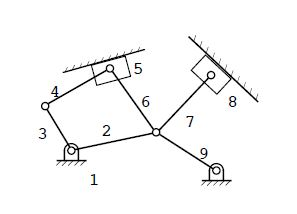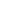### الصفحاتم3 نظرية الالات مرحلة ثالثة

# Theory of Machines Solutions to Chapter 1 Exercise Problems حلول الالات الفصل الاول

## Solutions to Chapter 1 Exercise Problems

Problem 1.1

Find a mechanism as an isolated device or in a machine and make a realistic sketch of the mechanism.  Then make a freehand sketch of the kinematic schematics for the mechanism chosen. Typical examples of solutions for this problem are given in the problem definitions of Chapter 3. Some examples are:

Problem 1.2

Cabinet hinges use various types of linkages for the folding mechanism.  Identify three types of cabinet hinges and make a freehand sketch of the kinematic mechanism used. There are a large number of mechanisms that are used to obtain various types of hinge motions. Below are three of them.  The first is a 6-bar Watt’s linkage used for chest lids.  The hinge guides the chest lid such that no part of the lid crosses the plane of the back of the chest.  The second example is a four bar linkage that guides the door from the open to closed position.  The hinge is basically hidden when the door is closed.  The third uses a 6-bar Watt’s linkage with a slider.  The lid glides about the back corner of the box.

Problem 1.3

The drawings shown below are pictorial representations of real mechanisms that are commonly encountered.   Make  a  freehand sketch  of  the  kinematic schematic representation of  each mechanism.

Problem 1.4

Linkages are often used to guide devices such as computer keyboards in and out of cabinets.  Find three such devices, and make a freehand sketch of the kinematic mechanisms used for the devices.

Problem 1.5

Fourbar linkages are used in common devices around the home and businesses.  Locate six such devices and make a freehand sketch of each device and describe its function. Solution:  Sample examples are given in the following:

Problem 1.6

Calculate the mobility, or number of degrees of freedom, of each of the mechanisms in Problem 1.3.

Problem 1.7

What is the number of members, number of joints, and mobility of each of the planar linkages shown below?

Problem 1.8

What are the number of members, number of joints, and mobility of each of the planar linkages shown below?

Problem 1.9

Determine the mobility and the number of idle degrees of freedom of each of the planar linkages shown below.  Show the equations used and identify the input and output links assumed when determining your answers.

Problem 1.10

Determine the mobility and the number of idle degrees of freedom of the linkages shown below. Show the equations used and identify any assumptions made when determining your answers.

Problem 1.11

Determine the mobility and the number of idle degrees of freedom associated with the mechanism. Show the equations used and identify any assumptions made when determining your answers.

Problem 1.12

Determine the mobility of each of the planar linkages shown below.  Show the equations used to determine your answers.

Problem 1.13

Determine the mobility and the number of idle degrees of freedom of each of the planar linkages shown below.  Show the equations used to determine your answers.

Problem 1.14

Determine the mobility and the number of idle degrees of freedom of each of the planar linkages shown below.  Show the equations used to determine your answers.

Problem 1.15

Determine the mobility and the number of idle degrees of freedom of each of the planar linkages shown below.  Show the equations used to determine your answers.

Problem 1.16

If position information is available for all points in the planar linkage shown below, can all of the velocities be determined uniquely if the value of  is given?  Explain your answer.

Problem 1.17

Determine the mobility and the number of idle degrees of freedom associated with each mechanism. Show the equations used and identify any assumptions made when determining your answers.

Problem 1.181

Determine the mobility and the number of idle degrees of freedom associated with the mechanism shown below.  The mechanism is a side-dumping car that consists of body 2 and truck 3 connected together by two six-bar linkages, ABCDEF and AGHKLMN.  Link NM is designed as a latch on its free end (see left drawing).  When jack 1 is operated, body 3 is lifted to the dumping position shown in the right-hand drawing.  Simultaneously, the six-bar linkage AGHKLMN opens the latch on link  NM and raises link GH.  Linkage ABCDEF swings open side BC and the load can be dumped at some distance from the car (see right-hand drawing).  Show the equations used to determine your answers.

Problem 1.19

Determine the mobility and the number of idle degrees of freedom associated with the mechanism below.  The round part rolls without slipping on the pieces in contact with it.

Problem 1.20

Determine the mobility and the number of idle degrees of freedom for each of the mechanisms shown. Show the equations used to determine your answers.

Problem 1.21

Determine the mobility and the number of idle degrees of freedom for each of the mechanisms shown.  Show the equations used and identify any assumptions made when determining your answers.

Problem 1.22

Determine the mobility and the number of idle degrees of freedom associated with the mechanism below.  The figure is a schematic of the entire linkage for a large power shovel used in strip mining. It can cut into a bank 20 m high and can dump to a height of 14.5 m.  Link 7 is connected to link 8 with a revolute joint.

Problem 1.23

In the figure is a portion of the support mechanism for the dipper on a large earth-moving machine used in removing overburden in strip mining operations.  The fixed centers for the portion of the mechanism really move, but useful information can be obtained by observing the dipper motion relative to the "frame" as shown in the sketch.  Both links 4 and 5 are mounted at O4.  Links 4 and 6 are parallel and of equal length.  The dipper is moved by a hydraulic cylinder driving crank 5 about its fixed cylinder.  Determine the number of degrees of freedom of the mechanism.

Problem 1.24

What is the number of members, number of joints, mobility, and the number of idle degrees of freedom  of each of the spatial linkages shown below?

Problem 1.25

Determine the mobility and the number of idle degrees of freedom of the spatial linkages shown below.  Show the equations used to determine your answers.

Problem 1.26

Determine the mobility and the number of idle degrees of freedom of the spatial linkages shown below.  Show the equations used to determine your answers.

Problem 1.27

Determine the mobility and the number of idle degrees of freedom for each of the mechanisms shown. Show the equations used to determine your answers.

Problem 1.28

Determine the mobility and the number of  idle  degrees of  freedom associated with each mechanism.3  Show the equations used to determine your answers.

Problem 1.29

Determine the mobility and the number of idle degrees of freedom for each of the mechanisms shown.  Show the equations used to determine your answers.  For the idle degrees of freedom, identify the input and output links assumed.

Problem 1.30

Determine which (if either) of the following linkages can be driven by a constant-velocity motor. For the linkage(s) that can be driven by the motor, indicate the driver link.

Problem 1.31

Assume that you have a set of links of the following lengths:  2 in, 4 in, 5 in, 6 in, 9 in.  Design a 4- bar linkage that can be driven with a continuously rotating electric motor.  Justify your answer with appropriate equations, and make a scaled drawing of the linkage.  Label the crank, frame, coupler, and rocker (follower). Coupler

Problem 1.32

Assume that you have a set of links of the following lengths: 20 mm, 30 mm, 45 mm, 56 mm, 73 mm.  Design a four-bar linkage that can be driven with a continuous-rotation electric motor.  Justify your answer with appropriate equations, and make a freehand sketch (labeled) of the resulting linkage.  Label the crank, frame, coupler, and rocker (follower).

Problem 1.33

For the four-bar linkages below, indicate whether they are Grashof type 1 or 2 and whether they are crank-rocker, double-crank, or double-rocker mechanisms.

Problem 1.34

You are given a set of three links with lengths 2.4 in, 7.2 in, and 3.4 in.  Select the length of a fourth link and assemble a linkage that can be driven by a continuously rotating motor.  Is your linkage a Grashof type 1 or Grashof type 2 linkage?  (Show your work.)  Is it a crank-rocker, double-rocker, or double-crank linkage?  Why?

Problem 1.35

You have available a set of eight links from which you are to design a four-bar linkage.  Choose the links such that the linkage can be driven by a continuous-rotation motor.  Sketch the linkage and identify the type of four-bar mechanism resulting.

Problem 1.36

Determine the number of fully rotating cranks in the planar mechanisms shown below.  Show your calculations.

Problem 1.37

If the link lengths of a four-bar linkage are L1 = 1 mm, L2 = 3 mm, L3 = 4 mm, and L4 = 5 mm and link 1 is fixed, what type of four-bar linkage is it?  Also, is the linkage a Grashof type 1 or 2 linkage?  Answer the same questions if L1 = 2 mm.

Problem 1.38

You are given two sets of links.  Select four links from each set such that the coupler can rotate fully with respect to the others.  Sketch the linkage and identify the type of four-bar mechanism.

Problem 1.39

The mechanisms shown below are drawn to scale. (a)  Sketch kinematic schematics showing the relationships between the members and joints. (b)  Determine the Grashof type of each four-bar linkage in each mechanism.

Problem 1.14

Determine the mobility and the number of idle degrees of freedom for each of the mechanisms shown.  Show the equations used to determine your answers.

Problem 1.16

Determine the mobility and the number of idle degrees of freedom associated with each mechanism. Show the equations used to determine your answers.